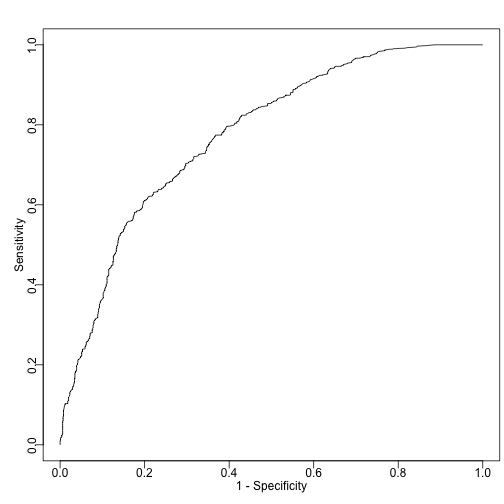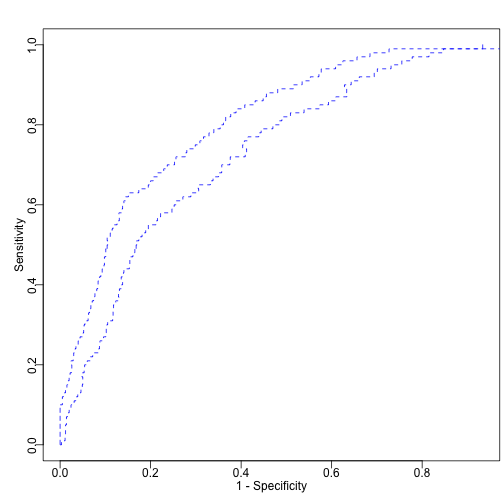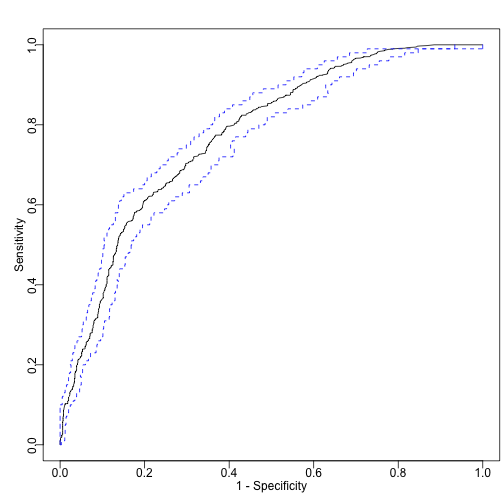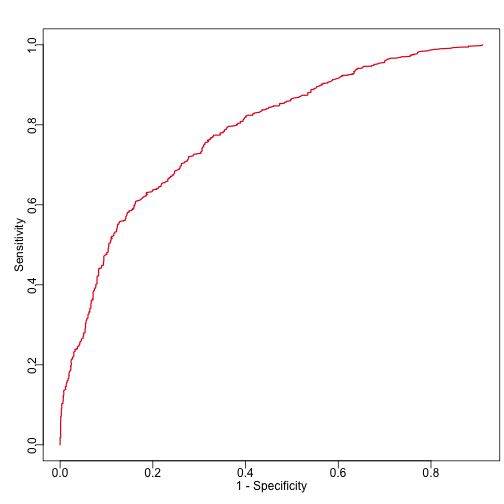`caROC` is an R package devoted to the assessment of continuous biomarkers. The metrics considered include specificity at contolled sensitivity level, sensitivity at controlled specificity level, and receiver operating characteristic (ROC) curve. If evaluation in specific sub-population is interested, all these statistics can also be computed in the version sub-population specific analysis. We allow both categorical and continuous covariates to be adjusted in computing these metrics.

## Installation and quick start

### Install caROC

Install `caROC` through

``````library(devtools)
install_github("ziyili20/caROC")
``````

### How to get help for caROC

Any caROC questions should be posted to the GitHub Issue section of caROC homepage at https://github.com/ziyili20/caROC/issues.

### Quick start on evaluating continuous biomarker with covariates adjusted

``````library(caROC)
## get specificity at controlled sensitivity levels 0.2, 0.8, 0.9
caROC(diseaseData,controlData,formula,
control_sensitivity = c(0.2,0.8, 0.9),
control_specificity = NULL)

## get covariate-adjusted ROC curve with curve-based monotonizing method
curveROC <- caROC(diseaseData,controlData,formula,
mono_resp_method = "curve",
verbose = FALSE)
``````

### Illustrating the usage of caROC in details

The tutorial is based on a simulation dataset:

``````library(caROC)
### n1: number of cases
### n0: number of controls
n1 = n0 = 1000

## Z_D and Z_C are the covariates in the disease and control groups
Z_D1 <- rbinom(n1, size = 1, prob = 0.3)
Z_D2 <- rnorm(n1, 0.8, 1)

Z_C1 <- rbinom(n0, size = 1, prob = 0.7)
Z_C2 <- rnorm(n0, 0.8, 1)

Y_C_Z0 <- rnorm(n0, 0.1, 1)
Y_D_Z0 <- rnorm(n1, 1.1, 1)
Y_C_Z1 <- rnorm(n0, 0.2, 1)
Y_D_Z1 <- rnorm(n1, 0.9, 1)

## M0 and M1 are the outcome of interest (biomarker to be evaluated) in the control and disease groups
M0 <- Y_C_Z0 * (Z_C1 == 0) + Y_C_Z1 * (Z_C1 == 1) + Z_C2
M1 <- Y_D_Z0 * (Z_D1 == 0) + Y_D_Z1 * (Z_D1 == 1) + 1.5 * Z_D2

diseaseData <- data.frame(M = M1, Z1 = Z_D1, Z2 = Z_D2)
controlData <- data.frame(M = M0, Z1 = Z_C1, Z2 = Z_C2)

## we are interested in evaluating biomarker M while adjusting for covariate Z
userFormula = "M~Z1+Z2"
``````

## 1. Covariate-adjusted sensitivity at controlled specificity level (or the reverse)

### 1.1 Compute pooled sensitivity at controlled specificed level

One can easily compute covariate-adjusted specificity at controlled sensitivity levels by specifying `control_sensitivity` and leaving `control_specificity` NULL.

`mono_resp_method` is to choose which monotonicity restoration method to use, “none” or “ROC”. `whichSE` is to choose how to compute standard error. It could be “boostrap” or “numerical”, i.e. boostrap-based or sample-based SE. Try ?caROC to see more details of these arguments.

``````caROC(diseaseData,controlData,userFormula,
control_sensitivity = c(0.2,0.8, 0.9),
control_specificity = NULL,
mono_resp_method = "ROC",
whichSE = "bootstrap",nbootstrap = 100,
CI_alpha = 0.95, logit_CI = TRUE)
#> \$Estimate
#>  0.959119 0.588000 0.428000
#>
#> \$SE
#>  0.008477755 0.030456890 0.025500792
#>
#> \$ConfidenceInterval
#>                     0.2       0.8       0.9
#> LogitCI_Lower 0.9388643 0.5272961 0.3789113
#> LogitCI_Upper 0.9728572 0.6461398 0.4785482
``````

To compute covariate-adjusted sensitivity at controlled specificity levels by specifying `control_specificity` and leaving `control_sensitivity` NULL.

``````caROC(diseaseData,controlData,userFormula,
control_sensitivity = NULL,
control_specificity = c(0.7,0.8, 0.9),
mono_resp_method = "none",
whichSE = "sample",nbootstrap = 100,
CI_alpha = 0.95, logit_CI = TRUE)
#> \$Estimate
#>  0.773 0.642 0.480
#>
#> \$SE
#>  0.01742042 0.02521579 0.02277279
#>
#> \$ConfidenceInterval
#>                     0.7       0.8       0.9
#> LogitCI_Lower 0.7370608 0.5912230 0.4356429
#> LogitCI_Upper 0.8053244 0.6897792 0.5246746
``````

### 1.2 Sub-population specific sensitivity at controlled specificity level

Give the covariates of a subpopulation, we can also computed sensitivity at controlled specificity level.

``````target_covariates = c(1, 0.7, 0.9)

sscaROC(diseaseData,controlData,
userFormula = userFormula,
control_sensitivity = c(0.2,0.8, 0.9),
target_covariates = target_covariates,
control_specificity = NULL,
mono_resp_method = "none",
whichSE = "sample",nbootstrap = 100,
CI_alpha = 0.95, logit_CI = TRUE)
#> \$Estimate
#>  0.9755622 0.6176939 0.4348163
#>
#> \$SE
#>  0.004991417 0.031733086 0.016981391
#>
#> \$ConfidenceInterval
#>                     0.2       0.8       0.9
#> LogitCI_Lower 0.9636149 0.5538888 0.4018745
#> LogitCI_Upper 0.9836531 0.6776837 0.4683440
``````

You can also specific covariates for multiple subpopualtions:

``````target_covariates = matrix(c(1, 0.7, 0.9,
1, 0.8, 0.8), 2, 3, byrow = TRUE)
sscaROC(diseaseData,controlData,
userFormula = userFormula,
control_sensitivity = c(0.2,0.8, 0.9),
target_covariates = target_covariates,
control_specificity = NULL,
mono_resp_method = "none",
whichSE = "sample",nbootstrap = 100,
CI_alpha = 0.95, logit_CI = TRUE)
#> \$Estimate
#> \$Estimate\$`1_0.7_0.9`
#>  0.9712095 0.5786200 0.3962791
#>
#> \$Estimate\$`1_0.8_0.8`
#>  0.9712095 0.5786200 0.3962791
#>
#>
#> \$SE
#> \$SE\$`1_0.7_0.9`
#>  0.005433995 0.035956544 0.017510216
#>
#> \$SE\$`1_0.8_0.8`
#>  0.005433995 0.035956544 0.017510216
#>
#>
#> \$ConfidenceInterval
#> \$ConfidenceInterval\$`1_0.7_0.9`
#>                     0.2       0.8       0.9
#> LogitCI_Lower 0.9584174 0.5070172 0.3625199
#> LogitCI_Upper 0.9801479 0.6470624 0.4310563
#>
#> \$ConfidenceInterval\$`1_0.8_0.8`
#>                     0.2       0.8       0.9
#> LogitCI_Lower 0.9584174 0.5070172 0.3625199
#> LogitCI_Upper 0.9801479 0.6470624 0.4310563
``````

### 2.1 Pooled ROC

Obtaining the covariate-adjusted ROC curve with sensitivity controlled through the whole spectrum is very easy. You can choose restoring monotonicity or no restoration when constructing ROC through argument `mono_resp_method`. It could be “none” (no monotonicity restoration) or “ROC” (curve-based monotonicity restoration).

``````### ROC with curve-based monotonicity restoration
curveROC <- caROC(diseaseData,controlData,userFormula,
mono_resp_method = "ROC",
verbose = FALSE)
``````

Plot the ROC curves:

``````oldpar <- par()
par(mar = c(3, 3, 2, 0.3), mgp = c(1.2, 0.3, 0))
plot_caROC(curveROC)
````````````par(oldpar)
``````

Construct confidence-band for the ROC curve:

``````curveROC_CB <- caROC_CB(diseaseData,controlData,
userFormula,
mono_resp_method = "ROC",
CB_alpha = 0.95,
nbin = 100,verbose = FALSE)
``````

Plot the confidence band:

``````oldpar <- par()
par(mar = c(3, 3, 2, 0.3), mgp = c(1.2, 0.3, 0))
plot_caROC_CB(curveROC_CB, add = FALSE, lty = 2, col = "blue")
````````````par(oldpar)
``````

or plot the ROC and confidence band on the same plot:

``````oldpar <- par()
par(mar = c(3, 3, 2, 0.3), mgp = c(1.2, 0.3, 0))
plot_caROC(curveROC)
plot_caROC_CB(curveROC_CB, add = TRUE, lty = 2, col = "blue")
````````````par(oldpar)
``````

### 2.2 Sub-population specific ROC

The ROC curve for given subpopulation can be easily calculated:

``````target_covariates = c(1, 0.7, 0.9)
myROC <- sscaROC(diseaseData,
controlData,
userFormula,
target_covariates,
global_ROC_controlled_by = "sensitivity",
mono_resp_method = "none")
oldpar <- par()
par(mar = c(3, 3, 2, 0.3), mgp = c(1.2, 0.3, 0))
plot_sscaROC(myROC, lwd = 1.6)
````````````par(oldpar)
``````

Confidence band can also be computed, but may take ~10-20min for a dataset with 2000 samples.

``````myROCband <- sscaROC_CB(diseaseData,
controlData,
userFormula,
mono_resp_method = "none",
target_covariates,
global_ROC_controlled_by = "sensitivity",
CB_alpha = 0.95,
logit_CB = FALSE,
nbootstrap = 100,
nbin = 100,
verbose = FALSE)
oldpar <- par()
par(mar = c(3, 3, 2, 0.3), mgp = c(1.2, 0.3, 0))
plot_sscaROC_CB(myROCband, col = "purple", lty = 2)
par(oldpar)
``````

## 3. Threshold at controlled sensitivity/specificity for given covariate values

In clinical setting, it is useful to know the specific thresholds of biomarkers at controlled sensitivity or specificity level for given covariate values.

``````### this is the given covariates of interest
new_covariates <- data.frame(M = 1,
Z1 = 0.7,
Z2 = 0.9)
### controlling sensitivity levels
caThreshold(userFormula, new_covariates,
diseaseData = diseaseData,
controlData = NULL,
control_sensitivity = c(0.7,0.8,0.9),
control_specificity = NULL)
#>   control_sens=0.7 control_sens=0.8 control_sens=0.9
#> 1         1.738084         1.371345        0.9148011

### controlling specificity levels
caThreshold(userFormula,new_covariates,
diseaseData = NULL,
controlData = controlData,
control_sensitivity = NULL,
control_specificity = c(0.7,0.8,0.9))
#>   control_spec=0.7 control_spec=0.8 control_spec=0.9
#> 1         1.584796         1.945257         2.409598
``````